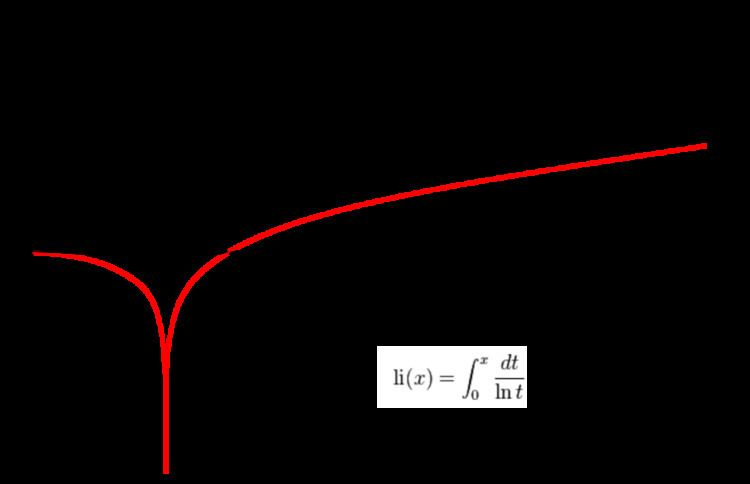# Logarithmic integral function

Updated onIn mathematics, the logarithmic integral function or integral logarithm li(x) is a special function. It is relevant in problems of physics and has number theoretic significance, occurring in the prime number theorem as an estimate of the number of prime numbers less than a given value.

## Integral representation

The logarithmic integral has an integral representation defined for all positive real numbers x ≠ 1 by the definite integral

l i ( x ) = 0 x d t ln t   .

Here, ln denotes the natural logarithm. The function 1/ln(t) has a singularity at t = 1, and the integral for x > 1 has to be interpreted as a Cauchy principal value,

l i ( x ) = lim ε 0 + ( 0 1 ε d t ln t + 1 + ε x d t ln t )   .

## Offset logarithmic integral

The offset logarithmic integral or Eulerian logarithmic integral is defined as

L i ( x ) = l i ( x ) l i ( 2 )

or, integrally represented

L i ( x ) = 2 x d t ln t

As such, the integral representation has the advantage of avoiding the singularity in the domain of integration.

This function is a very good approximation to the number of prime numbers less than x.

## Special values

The function li(x) has a single positive zero; it occurs at x ≈ 1.45136 92348 ...  A070769; this number is known as the Ramanujan–Soldner constant.

li(2) ≈ 1.045163 780117 492784 844588 889194 613136 522615 578151…  A069284

This is ( Γ ( 0 , ln 2 ) + i π ) where Γ ( a , x ) is the incomplete gamma function. It must be understood as the Cauchy principal value of the function.

## Series representation

The function li(x) is related to the exponential integral Ei(x) via the equation

li ( x ) = Ei ( ln x ) ,

which is valid for x > 0. This identity provides a series representation of li(x) as

l i ( e u ) = Ei ( u ) = γ + ln | u | + n = 1 u n n n !  for  u 0 ,

where γ ≈ 0.57721 56649 01532 ...  A001620 is the Euler–Mascheroni gamma constant. A more rapidly convergent series due to Ramanujan is

l i ( x ) = γ + ln ln x + x n = 1 ( 1 ) n 1 ( ln x ) n n ! 2 n 1 k = 0 ( n 1 ) / 2 1 2 k + 1 .

## Asymptotic expansion

The asymptotic behavior for x → ∞ is

l i ( x ) = O ( x ln x ) .

where O is the big O notation. The full asymptotic expansion is

l i ( x ) x ln x k = 0 k ! ( ln x ) k

or

l i ( x ) x / ln x 1 + 1 ln x + 2 ( ln x ) 2 + 6 ( ln x ) 3 + .

This gives the following more accurate asymptotic behaviour:

l i ( x ) x ln x = O ( x ln 2 x ) .

Note that, as an asymptotic expansion, this series is not convergent: it is a reasonable approximation only if the series is truncated at a finite number of terms, and only large values of x are employed. This expansion follows directly from the asymptotic expansion for the exponential integral.

## Number theoretic significance

The logarithmic integral is important in number theory, appearing in estimates of the number of prime numbers less than a given value. For example, the prime number theorem states that:

π ( x ) Li ( x )

where π ( x ) denotes the number of primes smaller than or equal to x .

## References

Similar Topics
The Marshal of Finland
Rosalind Picard
Kishor Gurung
Topics